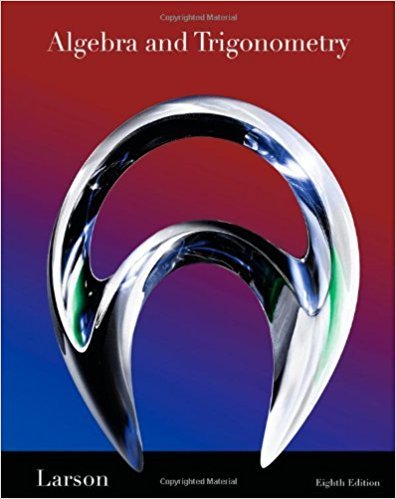×
×

# Solutions for Chapter 6.1: Angles and Their Measure## Full solutions for Algebra and Trigonometry | 8th Edition

ISBN: 9781439048474Solutions for Chapter 6.1: Angles and Their Measure

Solutions for Chapter 6.1
4 5 0 289 Reviews
18
2
##### ISBN: 9781439048474

Algebra and Trigonometry was written by and is associated to the ISBN: 9781439048474. Since 124 problems in chapter 6.1: Angles and Their Measure have been answered, more than 48701 students have viewed full step-by-step solutions from this chapter. This expansive textbook survival guide covers the following chapters and their solutions. This textbook survival guide was created for the textbook: Algebra and Trigonometry, edition: 8. Chapter 6.1: Angles and Their Measure includes 124 full step-by-step solutions.

Key Math Terms and definitions covered in this textbook
• Cayley-Hamilton Theorem.

peA) = det(A - AI) has peA) = zero matrix.

• Cofactor Cij.

Remove row i and column j; multiply the determinant by (-I)i + j •

• Determinant IAI = det(A).

Defined by det I = 1, sign reversal for row exchange, and linearity in each row. Then IAI = 0 when A is singular. Also IABI = IAIIBI and

• Dot product = Inner product x T y = XI Y 1 + ... + Xn Yn.

Complex dot product is x T Y . Perpendicular vectors have x T y = O. (AB)ij = (row i of A)T(column j of B).

• Eigenvalue A and eigenvector x.

Ax = AX with x#-O so det(A - AI) = o.

• Fibonacci numbers

0,1,1,2,3,5, ... satisfy Fn = Fn-l + Fn- 2 = (A7 -A~)I()q -A2). Growth rate Al = (1 + .J5) 12 is the largest eigenvalue of the Fibonacci matrix [ } A].

• Fundamental Theorem.

The nullspace N (A) and row space C (AT) are orthogonal complements in Rn(perpendicular from Ax = 0 with dimensions rand n - r). Applied to AT, the column space C(A) is the orthogonal complement of N(AT) in Rm.

• Hermitian matrix A H = AT = A.

Complex analog a j i = aU of a symmetric matrix.

• Incidence matrix of a directed graph.

The m by n edge-node incidence matrix has a row for each edge (node i to node j), with entries -1 and 1 in columns i and j .

• Least squares solution X.

The vector x that minimizes the error lie 112 solves AT Ax = ATb. Then e = b - Ax is orthogonal to all columns of A.

• Linear transformation T.

Each vector V in the input space transforms to T (v) in the output space, and linearity requires T(cv + dw) = c T(v) + d T(w). Examples: Matrix multiplication A v, differentiation and integration in function space.

• Markov matrix M.

All mij > 0 and each column sum is 1. Largest eigenvalue A = 1. If mij > 0, the columns of Mk approach the steady state eigenvector M s = s > O.

• Partial pivoting.

In each column, choose the largest available pivot to control roundoff; all multipliers have leij I < 1. See condition number.

• Random matrix rand(n) or randn(n).

MATLAB creates a matrix with random entries, uniformly distributed on [0 1] for rand and standard normal distribution for randn.

• Row picture of Ax = b.

Each equation gives a plane in Rn; the planes intersect at x.

• Simplex method for linear programming.

The minimum cost vector x * is found by moving from comer to lower cost comer along the edges of the feasible set (where the constraints Ax = b and x > 0 are satisfied). Minimum cost at a comer!

• Skew-symmetric matrix K.

The transpose is -K, since Kij = -Kji. Eigenvalues are pure imaginary, eigenvectors are orthogonal, eKt is an orthogonal matrix.

• Spanning set.

Combinations of VI, ... ,Vm fill the space. The columns of A span C (A)!

• Symmetric factorizations A = LDLT and A = QAQT.

Signs in A = signs in D.

• Trace of A

= sum of diagonal entries = sum of eigenvalues of A. Tr AB = Tr BA.

×